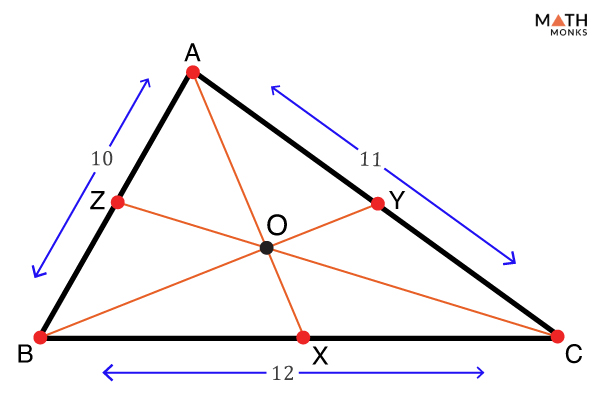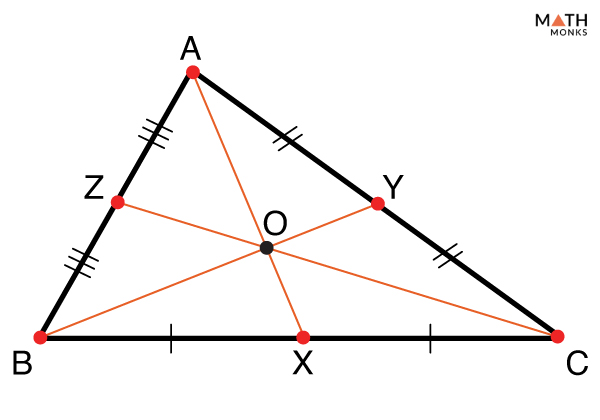# Ceva’s Theorem

Ceva’s theorem is a theorem related to triangles in Euclidean plane geometry. It provides the condition for a triangle’s concurrent cevians (lines from vertex to any point on the opposite side of that vertex).

## Statement

Let us consider a △ABC and let X, Y, and Z be the points on the sides BC, CA, and AB, respectively.

Then according to Ceva’s theorem, three lines (cevians) AX, BY, and CZ joining vertices A, B, and C to there opposite sides will be concurrent if ${\dfrac{BX}{XC}}$ × ${\dfrac{CY}{YA}}$ × ${\dfrac{AZ}{ZB}}$ = 1.

## Proof

We draw a △ABC with the points X, Y, and Z on BC, CA, and AB respectively. Also, let the lines AX, BY, and CZ be concurrent. Though the point of concurrency may lie inside or outside the triangle, we draw it (point O) inside the triangle.

Ceva’s theorem can be proved in various ways.

### Using Triangle’s Area

The notation [ABC] denotes the area of a triangle with vertices A, B, and C.

Step 1:

△ABX and △AXC have the same altitude AP but bases BX and XC.

Therefore [ABX] = ${\dfrac{1}{2}}$ × BX × AP ……….(i) and

[AXC] = ${\dfrac{1}{2}}$ × XC × AP ……….(ii)

Dividing (i) by (ii) gives ${\dfrac{[ABX]}{[AXC]}}$ = ${\dfrac{BX}{XC}}$ ……….(iii)

Step 2:

Let us draw another line OM joining O and BC perpendicular to BC. △OBX and △OXC have the same altitude OM but bases BX and XC.

Hence, [OBX] = ${\dfrac{1}{2}}$ × BX × OM ……….(iv) and

[OXC] = ${\dfrac{1}{2}}$ × XC × OM ……….(v)

Again dividing (iv) by (v) gives ${\dfrac{[OBX]}{[OXC]}}$ = ${\dfrac{BX}{XC}}$ ……….(vi)

Step 3: from (iii) and (vi), we get,

${\dfrac{[ABX]}{[AXC]}}$ = ${\dfrac{[OBX]}{[OXC]}}$ = ${\dfrac{BX}{XC}}$

Or, ${\dfrac{[ABX] – [OBX]}{[AXC] – [OXC]}}$ = ${\dfrac{BX}{XC}}$

Or, ${\dfrac{[AOB]}{[AOC]}}$ = ${\dfrac{BX}{XC}}$ ……….(vii)

Step 4: Similarly, we get ${\dfrac{[BOC]}{[AOB]}}$ = ${\dfrac{CY}{YA}}$ ……….(viii) and

${\dfrac{[AOC]}{[BOC]}}$ = ${\dfrac{AZ}{ZB}}$ ……….(ix)

Step 5: Multiplying (vii), (viii), and (ix) we have,

${\dfrac{[AOB]}{[AOC]}}$ × ${\dfrac{[BOC]}{[AOB]}}$ × ${\dfrac{[AOC]}{[BOC]}}$ = ${\dfrac{BX}{XC}}$ × ${\dfrac{CY}{YA}}$ × ${\dfrac{AZ}{ZB}}$ = 1

Hence the Ceva’s theorem is proved.

### Using Similar Triangles

Let us draw a line through A and parallel to BC. BY and CZ are extended beyond the triangle to intersect the newly drawn parallel line at points N and M, respectively.

Step 1:

For △AMZ and △ZBC,

∠MAZ = ∠ZBC = ∠1 [alternate interior angles]

∠AMZ = ∠ZCB = ∠2 [alternate interior angles]

So, ∠AZM = ∠BZC =∠3

Hence △AMZ and △ZBC are similar triangles and

${\dfrac{AZ}{ZB}}$ = ${\dfrac{AM}{BC}}$ = ${\dfrac{MZ}{ZC}}$ ……….(i)

Step 2: There are 3 more pairs of similar triangles:

△AMO and △OXC are similar triangles, so

${\dfrac{AM}{XC}}$ = ${\dfrac{AO}{OX}}$ = ${\dfrac{OM}{OC}}$ ……….(ii)

△NAO and △OXB are similar triangles, so

${\dfrac{AN}{BX}}$ = ${\dfrac{AO}{OX}}$ = ${\dfrac{NO}{BO}}$ ……….(iii)

△YBC and △ANY are also similar triangles, so

${\dfrac{BC}{AN}}$ = ${\dfrac{CY}{YA}}$ = ${\dfrac{BY}{YN}}$ ……….(iv)

Step 3: From (ii) and (iii), ${\dfrac{AO}{OX}}$ = ${\dfrac{AM}{XC}}$ = ${\dfrac{AN}{BX}}$,

i.e., ${\dfrac{BX}{XC}}$ = ${\dfrac{AN}{AM}}$ ……….(v)

Step 4: Using (i), (iv), and (v), we get

${\dfrac{AZ}{ZB}}$ × ${\dfrac{CY}{YA}}$ × ${\dfrac{BX}{XC}}$ = ${\dfrac{AM}{BC}}$ × ${\dfrac{BC}{AN}}$ × ${\dfrac{AN}{AM}}$ = 1

Hence the, Ceva’s theorem is proved.

### Using Barycentric Coordinates

Let the vertices A, B, and C of △ABC be (1, 0, 0), (0, 1, 0), and (0, 0, 1), respectively.

The barycentric coordinate (p1, p2, p3) of a point P inside △ABC will be given by

P = αA + βB + γC, where α + β + γ = 1

Step 1: As X lies on BC, the coordinate of X can be written as (0, t1, 1 – t1)

The equation of AX will be ${\dfrac{x – 1}{-1}}$ = ${\dfrac{y}{t_{1}}}$ = ${\dfrac{z}{1-t_{1}}}$

Considering the last two equalities we get, z = ${\dfrac{1-t_{1}}{t_{1}}}$ × y ……….(i)

Step 2 Step 2: Similarly, as Y and Z lie on AC and AB, respectively, we consider Y = (1 – t2, 0, t2) and Z = (t3, 1 – t3, 0)

Thus equation of BY: ${\dfrac{x}{1-t_{2}}}$ = ${\dfrac{y – 1}{-1}}$ = ${\dfrac{z}{t_{2}}}$

From the first and the last equalities, x = ${\dfrac{1-t_{2}}{t_{2}}}$ × z ……….(ii)

Equation of CZ: ${\dfrac{x}{t_{3}}}$ = ${\dfrac{y}{1-t_{3}}}$ = ${\dfrac{z}{-1}}$

From the first and the second equalities, y = ${\dfrac{1-t_{3}}{t_{3}}}$ × x ……….(iii)

Step 3: Multiplying (i), (ii), and (iii), we get,

x × y × z = ${\dfrac{1-t_{2}}{t_{2}}}$ × z × ${\dfrac{1-t_{3}}{t_{3}}}$ × x × ${\dfrac{1-t_{1}}{t_{1}}}$ × y

Dividing both sides by x × y × z gives

${\dfrac{1-t_{1}}{t_{1}}}$ × ${\dfrac{1-t_{2}}{t_{2}}}$ × ${\dfrac{1-t_{3}}{t_{3}}}$ = 1 ……….(iv)

Step 4: Now, finding the ratio at which X, Y, and Z intersect the sides BC, CA, and AB, respectively, we see that

${\dfrac{1-t_{1}}{t_{1}}}$ = ${\dfrac{BX}{XC}}$

${\dfrac{1-t_{2}}{t_{2}}}$ = ${\dfrac{CY}{YA}}$

${\dfrac{1-t_{3}}{t_{3}}}$ = ${\dfrac{AZ}{ZB}}$

Hence equation (iv) proves Ceva’s theorem.

## Converse of Ceva’sTheorem

Converse of Ceva’s theorem states that if for △ABC, X, Y, and Z are three points lying on the sides BC, CA, and AB, respectively, such that ${\dfrac{BX}{XC}}$ × ${\dfrac{CY}{YA}}$ × ${\dfrac{AZ}{ZB}}$ = 1, then the lines AX, BY, and CZ will be concurrent.

Step 1: Let the lines BY and CZ intersects at point O, and the line AO intersects BC at X’.

Then, according to Ceva’s theorem, ${\dfrac{BX’}{X’C}}$ × ${\dfrac{CY}{YA}}$ × ${\dfrac{AZ}{ZB}}$ = 1 ……….(i)

Step 2:Given, ${\dfrac{BX}{XC}}$ × ${\dfrac{CY}{YA}}$ × ${\dfrac{AZ}{ZB}}$ = 1 ……….(ii)

Step 3: Dividing (ii) by (i), we get,

${\dfrac{BX}{XC}}$ = ${\dfrac{BX’}{X’C}}$, which is possible if and only if X = X’.

Hence the converse of Ceva’s theorem is proved.

## Solved ExamplesSuppose AB, BC, and CA have lengths of 10, 11, and 12 units, respectively. If ${\dfrac{BX}{XC}}$ = ${\dfrac{3}{4}}$ and ${\dfrac{CY}{YA}}$ = ${\dfrac{4}{7}}$, then find AZ and ZB.

Solution:

According to Ceva’s theorem, ${\dfrac{BX}{XC}}$ × ${\dfrac{CY}{YA}}$ × ${\dfrac{AZ}{ZB}}$ = 1
So, putting the values of ${\dfrac{BX}{XC}}$ and ${\dfrac{CY}{YA}}$ we get, ${\dfrac{AZ}{ZB}}$ = ${\dfrac{7}{3}}$.
Let, ZB = x, then AZ = ${\dfrac{7x}{3}}$.
Therefore, AB = AZ + ZB = 10, i.e., ${\dfrac{10x}{3}}$ = 10.
Thus x = 3.
So, ZB = 3 units and AZ = 7 units.Show that if X, Y, and Z are the midpoints of the sides of a triangle, then the three cevians are concurrent.

Solution:

Let us consider a triangle, △ABC, and let X, Y, and Z be AB, BC, and CA midpoints, respectively.
Then, BX = XC, CY = YA and AZ = ZB
Hence, ${\dfrac{BX}{XC}}$ × ${\dfrac{CY}{YA}}$ × ${\dfrac{AZ}{ZB}}$ = 1., which satisfies the Ceva’s theorem.
Thus, the cevians AX, BY, and CZ are concurrent.